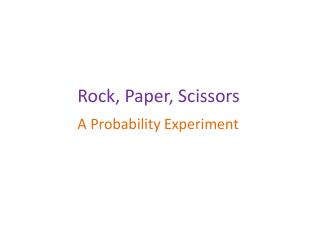DownloadDownload PresentationRock, Paper, Scissors

# Rock, Paper, Scissors

Download Presentation## Rock, Paper, Scissors

- - - - - - - - - - - - - - - - - - - - - - - - - - - E N D - - - - - - - - - - - - - - - - - - - - - - - - - - -
##### Presentation Transcript

1. Rock, Paper, Scissors A Probability Experiment

2. Probability is a measurement of the chance or likelihood of an event happening. The language of probability Words that we might use to describe probabilities include: 50-50 chance likely unlikely poor chance certain very likely possible impossible even chance probable

3. scissors paper rock In the game rock, paper,scissors two players have to show either rock, paper or scissors using their hands as follows: Rock, paper, scissors The rules of the game are that: Scissors beats paper (it cuts). Paper beats rock (it wraps). Rock beats scissors (it breaks). If both players show the same hands it is a draw.

4. Second player Scissors Paper Rock Scissors 1 3 First player 3 9 Paper Rock RR = SS PP What is the probability that both players will show the same hands in a game of rock, paper,scissors? We can list all the possible outcomes in a two-way table using R for Rock, P for Paper and S for Scissors. Rock, paper, scissors SP SR SS PS PP PR RS RP RR P(same hands) =

5. Second player Scissors Paper Rock SP SR SS Scissors 3 1 PS PP PR First player 9 3 Paper RS RP RR Rock RS = SP PR What is the probability that the first player will win a game of rock, paper,scissors? Using the two-way table we can identify all the ways that the first player can win. Rock, paper, scissors P(first player wins) =

6. Second player Scissors Paper Rock SP SR SS Scissors 3 1 PS PP PR First player 9 3 Paper RS RP RR Rock RP = SR PS What is the probability that the second player will win a game of rock,paper,scissors,? Using the two-way table we can identify all the ways that the second player can win. Rock, paper, scissors P(second player wins) =

7. P(first player wins) = P(second player wins) = P(a draw) = 1 1 1 3 3 3 Is rock, paper, scissors a fair game? Rock, paper, scissors Both player are equally likely to win so, yes, it is a fair game. Play rock,paper,scissors 30 times with a partner. Record the number of wins for each player and the number of draws. Are the results as you expected?

8. The number 18 has been drawn the most often in the lottery so I’m more likely to win if I choose it. I’ve just thrown four heads in a row so I’m much less likely to get a head on my next throw. There are two choices for lunch, pizza or pasta. That means that there is a 50% chance that the next person will choose pizza. I’m so unlucky. If I roll this dice I’ll never get a six. Statements involving probability are often incorrect or misleading. Discuss the following statements: Probability statements